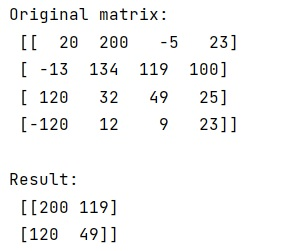# How to perform max/mean pooling on a 2d array using numpy?

Given a 2D NumPy array, we have to perform max/mean pooling on it.
Submitted by Pranit Sharma, on February 15, 2023

NumPy is an abbreviated form of Numerical Python. It is used for different types of scientific operations in python. Numpy is a vast library in python which is used for almost every kind of scientific or mathematical operation. It is itself an array which is a collection of various methods and functions for processing the arrays.

## Performing max/mean pooling on a 2d NumPy array

Suppose that we are given a 2D matrix and a 2D kernel and we need to return a matrix that is the result of max or mean pooling using the given kernel.

For this purpose, if the image size is evenly divisible by the kernel size, we can reshape the array and use max or mean as we see fit. If we do not have an even number of kernels, we have to handle the boundaries separately.

Let us understand with the help of an example,

## perform max/mean pooling on a 2d array using numpy

```# Import numpy
import numpy as np

# Creating a numpy matrix
mat = np.array([[  20,  200,   -5,   23],[ -13,  134,  119,  100],
[ 120,   32,   49,   25],
[-120,   12,   9,   23]])

# Display original matrix
print("Original matrix:\n",mat,"\n")

# Getting shape of matrix
M, N = mat.shape

# Shape of kernel
K = 2
L = 2

# Dividing the image size by kernel size
MK = M // K
NL = N // L

# Creating a pool
res = mat[:MK*K, :NL*L].reshape(MK, K, NL, L).max(axis=(1, 3))

# Display Result
print("Result:\n",res)
```

Output: